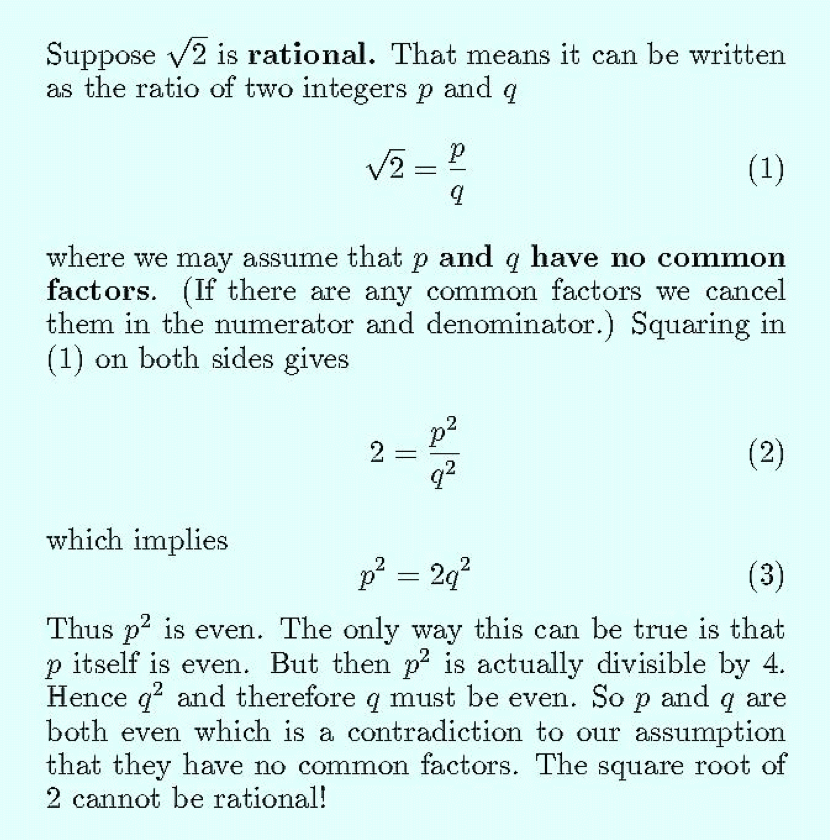### Oh! Triangle (The Square Root of Two Song)

Lyrics by Philip Carl
Sung to the tune of “Oh! Susanna” by Stephen Foster

If you take a right triangle
With sides a and b and c
Then “a” squared summed with “b” squared
Equals “c” squared don’t you see?

If a and b have unit length
When squared and summed it’s true
Your third side simply has to be
That strange square root of two

CHORUS
Oh! Triangle
What problems you let loose
If you are right then something’s wrong
With the hypotenuse

What is the length of that third side?
Oh how I wish I knew
It’s a number that when squared becomes
Precisely two

We write your value equal to
A “p” that’s over “q
So p squared over q squared
Must exactly equal two

Chorus

If p and q are integers
We write in lowest terms
A reduction to absurdity

For you can see that p is even
So indeed is q
So they can’t be in lowest terms
Which we assumed was true

Chorus

So the length of the hypotenuse
Is irrational you see
Which drove Pythagoras to drink
And sort of bothers me

Oh! Triangle
What problems you let loose
If you are right then something’s wrong
Yes something’s very very wrong
And I’ve tried to tell you in this song
Which has probably gone on much too long
With the hypotenuse!